# Graph, numerical characteristics of a

Functions specified on the set of graphs and taking values in a certain set of numbers. A number of such characteristics, with their most common notations, are given below. The simplest numerical characteristics of a graph are the number of vertices and the number of edges (arcs) of a graph. The cyclomatic number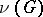ofis the smallest number of edges whose removal yields a graph without cycles: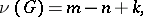whereis the number of edges,is the number of vertices andis the number of connected components of. The vertex-connectivity number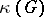(the edge-connectivity number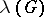) is the smallest number of vertices (edges) ofwhose removal yields a non-connected graph or a trivial graph (i.e. a graph consisting of a single vertex). The density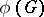is the largest number of vertices in a complete subgraph of; the independence number, or the number of internal stability,is the largest number of pairwise non-adjacent vertices of(and the pairwise non-adjacent vertices ofform an internally stable set). The chromatic number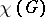(edge chromatic number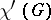) is the smallest number of colours required to colour the vertices (edges) ofso that any adjacent vertices (edges) are coloured with different colours (see also Graph colouring). The number of external stability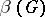is the smallest number of vertices in a subsetof the set of vertices ofsuch that any vertex not belonging tois adjacent to at least one vertex of. The aborescenceis the smallest number of spanning forests ofwhich do not intersect at the edges, and whose union yields. The coarseness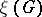is the largest number of non-planar subgraphs ofwhich do not intersect at the edges and whose union is. The thicknessis the smallest number of planar subgraphs whose union yields. The crossing number is the smallest number of pairwise intersections of the edges ofwhen placed on a plane. The genus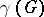ofis the smallest genus of the two-dimensional orientable surface on whichcan be placed without its edges intersecting (cf. Graph imbedding).
Certain numerical characteristics concern the number of subgraphs of the given graph of a certain type — e.g. the number of spanning trees, the number of Hamilton cycles, etc. There are characteristics which depend on a parameter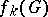(e.g. the number of complete subgraphs withvertices), and the totality of these characteristics can be specified by the polynomial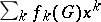— the analogue of the generating function. Many such polynomials can be found recursively by the application of operations on graphs — removal of a vertex or of an edge, contraction of an edge, etc. In solving graph-theoretic problems it is often necessary to study relationships between different numerical characteristics. These attain their extremal values on certain sets, and such values can often be used in describing the graphs on which they are attained. In such a case finding the extremal values is reduced to the study of such graphs. In studying the graphs for which a characteristic under consideration assumes a given value, it may be useful to study the properties of critical graphs (cf. Graph, extremal).